# 48+ Sample Metric Conversion Chart Templates

A metric conversion chart is a really important tool when you cannot fathom the true assessment of a particular metric unit. It could be that your weight is stated in kg while you understand it in pounds. In such situations, the metric conversion charts are really handy as they will offer you directly the converted metric of the original metric. There is a versatile range of metric conversion charts. Some deal with weight, some with length, some with temperature, some with distance and so on.

## >Metric System Conversion Chart Templates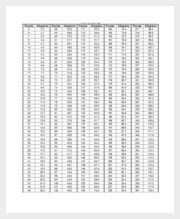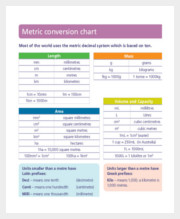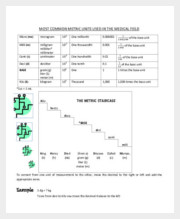A metric system conversion chart is a one where you have converted metrics of a particular attribute- like weight or distance or height and so on. If you are not comfortable with a certain metric unit, the conversion chart will come up with a converted unit beside the original unit for easier understanding. [8+ Metric System Conversion Chart Templates]

## > Simple Metric Conversion Chart Templates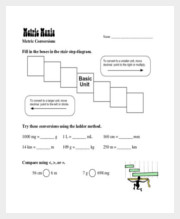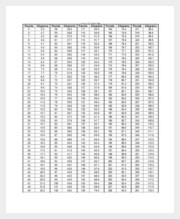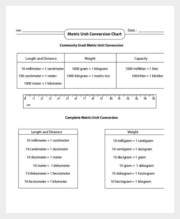A simple metric conversion chart will generally come up with 2 columns. On the left, you will have metric units and on the right, you will have customary units. Thus, if it’s cm, m, km, gm, l etc. on the left, at the right you will have the metrics converted into inches, feet, mile, gallon and so on. [8+ Simple Metric Conversion Chart Templates]

## > Basic Metric Conversion Chart Templates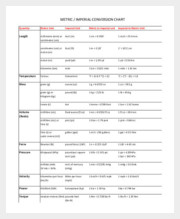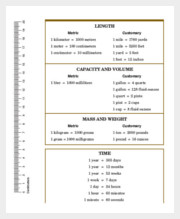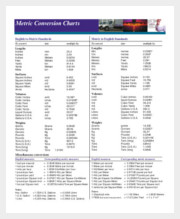A basic metric conversion chart generally deals with the conversion of metric units of one definite attribute. It could be area or length or volume or mass and so on. On one side you will have the one set of measurement units of that particular attribute and on another side, there would be another or multiple sets of metric expressions- to show you the converted measure. [9+ Basic Metric Conversion Chart Templates]

## > Metric Conversion Chart Templates for kids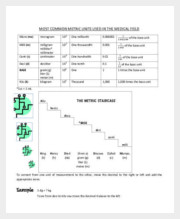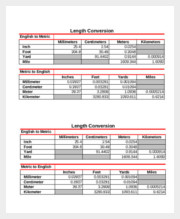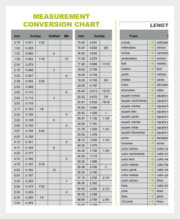There can be different metric conversion charts for kids to teach them the basics of different measurement units and to get them an idea of the varied metric systems. The most popular of these charts are charts for length and distance, capacity and weight. These are generally a part of the math course in the elementary classes. [8+ Metric Conversion Chart Templates For Kids]

## > Metric Weight Conversion Chart Templates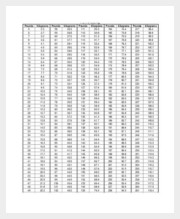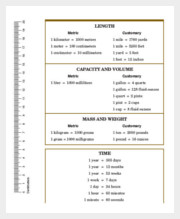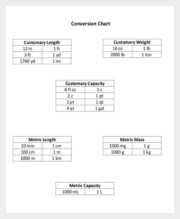Weight is expressed in a variety of measurement units such as kilograms, pounds, stones etc. If you are not really confident about a certain metric, you can always check out the metric weight conversion charts. These can be kilo to pounds or grams to ounce or kilo to stones or ounces to grams etc. [8+ Metric Weight Conversion Chart Templates]

## > Metric Unit Conversion Chart Templates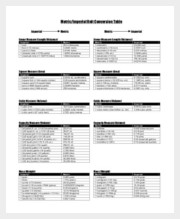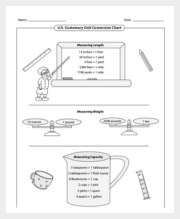There is a huge scale of metric unit conversion charts based on the specific unit you want to know about. It could be length or area or distance and so on. Some of the charts just provide one converted unit while the more elaborate ones can extend multiple converted metrics of one particular metric. [7+ Metric Unit Conversion Chart Templates]